# MCQs on Electrical Machines

##### Page 16 of 114. Go to page 1 2 3 4 5 6 7 8 9 10 11 12 13 14 15 16 17 18 19 20 21 22 23 24 25 26 27 28 29 30 31 32 33 34 35 36 37 38 39 40 41 42 43 44 45 46 47 48 49 50 51 52 53 54 55 56 57 58 59 60 61 62 63 64 65 66 67 68 69 70 71 72 73 74 75 76 77 78 79 80 81 82 83 84 85 86 87 88 89 90 91 92 93 94 95 96 97 98 99 100 101 102 103 104 105 106 107 108 109 110 111 112 113 114
01․ Which of the following motor is suitable for frequent starting and stoppings?
Capacitor start motor
Capacitor start capacitor run motor
Resistance split phase motor
All of the above.

In capacitor start motor, there is a capacitor in the starting winding through a centrifugal switch. When the stator is supplied with 1-phase supply the stator current, main winding current Im and starting winding current Is has a phase difference as near to 80° with the help of the capacitor and the motor is nearly a perfect two phase motor. This produces a rotating magnetic field and high starting torque with low starting current in the starting winding. Therefore capacitor start motor is preferred for frequent starting and stopping applications like refrigerators, grinders etc. The capacitor used for frequent starting is dry type electrolytic capacitor.

02․ The current drawn by the armature of DC motor is directly proportional to
The speed of motor.
The torque required.
The voltage across the terminals.
None of above.

In case of DC motor mechanical power developed by the armature = T × ω, where ω is the angular velocity in radian per second. Electrical equivalent of gross mechanical power developed by the armature (electromagnetic power) = Eb×Ia.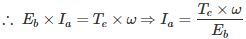∴ Ia ∝ Te (if back emf and angular velocity are constant)

03․ If the excitation is critical, the power factor of the alternator is
unity power factor.
lagging power factor.
none of the above.

Reactive power is given by the equation,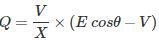From the above equation, the reactive power generated or delivered significantly depends on excitation. When excitation is rated or critical, E cosθ = V, which means Q = 0, the generator neither supplies nor draws any reactive power and operates at unity power factor.

04․ Maximum power developed depends on
voltage
reactance
both 1and 2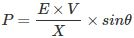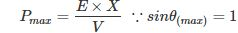Maximum power is developed when load angle θ = 90°. Therefore maximum power developed will depend only on voltage and reactance values.

05․ What is/are the advantages of parallel operation of alternators?
Increase in reliability
Increase efficiency under light load conditions
continuity supply is maintained
all of the above

The advantages of parallel operation of alternators are as follows:
1. Increase in reliability
2. Increase efficiency under light load conditions as the generators can be switched optimally according to the load.
3. Under maintenance or repair or routine work, continuity supply is maintained
4. Future expansion is quite easy
5. Any variation in voltage or frequency will hardly make a significant impact on the bus bar voltage and frequency.

06․ Which of the following relation is correct?
Xd = Xq.
Xd < Xq.
Xd > Xq.
None of the above.

Direct axis synchronous reactance Xd = Vmax / Imin Quadrature axis synchronous reactance Xq = Vmin / Imax Therefore, direct axis synchronous reactance Xd is greater than quadrature axis synchronous reactance Xq.

07․ What is/are the necessary conditions for parallel operation of alternators?
Terminal voltage is same
Frequency should be same
Speed of alternators should be same
Both 1and 2

Necessary conditions for parallel operation of alternators are as follows:
1. Terminal voltage is same
2. Frequency should be same
3. Phase sequence should be same

08․ Quadrature axis synchronous reactance is the ratio of
Vmax to Imax.
Vmin to Imax.
Vmax to Imin.
Vmin to Imin.

When the rotor and stator rotating magnetic field aligns along with the quadrature axis, air gap reluctance is maximum. Therefore more magnetizing current is drawn by the stator to maintain the flux and the voltage is minimum. ∴ Quadrature axis synchronous reactance Xq = Vmin / Imax.

09․ Direct axis reactance is defined as the ratio of
Vmax to Imax
Vmin to Imax
Vmax to Imin
Vmin to Imin

When rotor aligns with the stator rotating magnetic field along the direct axis the air gap reluctance is minimum and flux linkage is maximum and current drawn by the stator is minimum. Consequently the voltmeter and ammeter readings at that instant will be Vmax and Imin.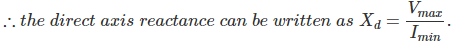10․ Voltage regulation can be determined by which of the following?
Synchronous impedance method.
MMf method.
ZPF method.
All of the above.

Indirect methods to find voltage regulation are as follows:
1. Synchronous impedance method.
2. MMF method (Magneto motive Force or Ampere turn method).
3. ZPF method (Zero Power Factor or potier triangle method).
4. American Standard Association Method (ASA method).

<<<1415161718>>>